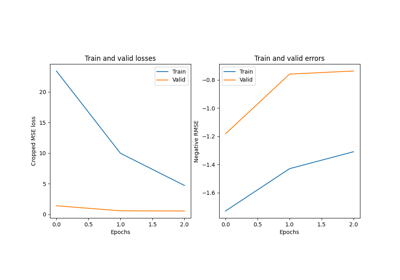# braindecode.models.Deep4Net#

class braindecode.models.Deep4Net(in_chans, n_classes, input_window_samples, final_conv_length, n_filters_time=25, n_filters_spat=25, filter_time_length=10, pool_time_length=3, pool_time_stride=3, n_filters_2=50, filter_length_2=10, n_filters_3=100, filter_length_3=10, n_filters_4=200, filter_length_4=10, first_conv_nonlin=<function elu>, first_pool_mode='max', first_pool_nonlin=<function identity>, later_conv_nonlin=<function elu>, later_pool_mode='max', later_pool_nonlin=<function identity>, drop_prob=0.5, split_first_layer=True, batch_norm=True, batch_norm_alpha=0.1, stride_before_pool=False)[source]#

Deep ConvNet model from Schirrmeister et al 2017.

Model described in [Schirrmeister2017].

Parameters
• in_chans (int) – Number of EEG input channels.

• n_classes (int) – Number of classes to predict (number of output filters of last layer).

• input_window_samples (int | None) – Only used to determine the length of the last convolutional kernel if final_conv_length is “auto”.

• final_conv_length (int | str) – Length of the final convolution layer. If set to “auto”, input_window_samples must not be None.

• n_filters_time (int) – Number of temporal filters.

• n_filters_spat (int) – Number of spatial filters.

• filter_time_length (int) – Length of the temporal filter in layer 1.

• pool_time_length (int) – Length of temporal pooling filter.

• pool_time_stride (int) – Length of stride between temporal pooling filters.

• n_filters_2 (int) – Number of temporal filters in layer 2.

• filter_length_2 (int) – Length of the temporal filter in layer 2.

• n_filters_3 (int) – Number of temporal filters in layer 3.

• filter_length_3 (int) – Length of the temporal filter in layer 3.

• n_filters_4 (int) – Number of temporal filters in layer 4.

• filter_length_4 (int) – Length of the temporal filter in layer 4.

• first_conv_nonlin (callable) – Non-linear activation function to be used after convolution in layer 1.

• first_pool_mode (str) – Pooling mode in layer 1. “max” or “mean”.

• first_pool_nonlin (callable) – Non-linear activation function to be used after pooling in layer 1.

• later_conv_nonlin (callable) – Non-linear activation function to be used after convolution in later layers.

• later_pool_mode (str) – Pooling mode in later layers. “max” or “mean”.

• later_pool_nonlin (callable) – Non-linear activation function to be used after pooling in later layers.

• drop_prob (float) – Dropout probability.

• split_first_layer (bool) – Split first layer into temporal and spatial layers (True) or just use temporal (False). There would be no non-linearity between the split layers.

• batch_norm (bool) – Whether to use batch normalisation.

• batch_norm_alpha (float) – Momentum for BatchNorm2d.

• stride_before_pool (bool) – Stride before pooling.

References

Schirrmeister2017

Schirrmeister, R. T., Springenberg, J. T., Fiederer, L. D. J., Glasstetter, M., Eggensperger, K., Tangermann, M., Hutter, F. & Ball, T. (2017). Deep learning with convolutional neural networks for EEG decoding and visualization. Human Brain Mapping , Aug. 2017. Online: http://dx.doi.org/10.1002/hbm.23730

## Examples using `braindecode.models.Deep4Net`#Regression example on fake data

Regression example on fake data